Springs: Hooke's Law & Elastic Limits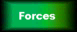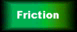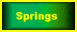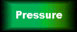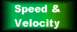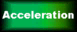## "Hooke's Law" is about stretching springs and wires.

When we apply a force to a spring, it stretches.

If we apply double the force, it stretches twice as much, so long as we don't over-do it.

So far, this is pretty obvious. Now let's look in more detail...

We measure the original length of the spring when we start. When it stretches, we measure the extension - that's how much longer it is than it was when we started.

Extension = present length – original length

If this picture isn't moving, click on "Refresh"/"Reload"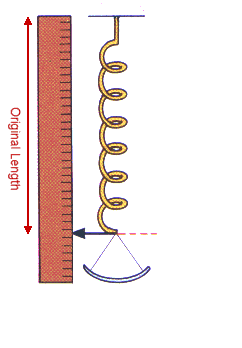Hooke's Law states:- the extension is proportional to the force the spring will go back to its original length when the force is removed so long as we don't exceed the elastic limit.

 The elastic limit is where the graph departs from a straight line. If we go past it, the spring won't go back to its original length. When we remove the force, we're left with a permanent extension. Below the elastic limit, we say that the spring is showing "elastic behaviour": the extension is proportional to the force, and it'll go back to it's original length when we remove the force. Beyond the elastic limit, we say that it shows "plastic behaviour". This means that when a force is applied to deform the shape, it stays deformed when the force is removed. We use Hooke's Law in spring balances, kitchen scales and other devices where we measure using a spring. We use a more complete version (called "Young's Modulus") when designing bridges, aircraft and other engineering projects. If this picture isn't moving, click on "Refresh"/"Reload"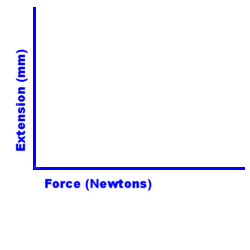Note: the point on the graph where it departs from a straight line is really called the "limit of proportionality". At GCSE level, for simple springs and wires, this can be considered to be the same as the elastic limit.

Spring Questions (click on the question to reveal the answers)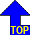of page